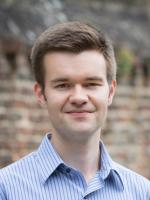skip to content

# Department of Pure Mathematics and Mathematical StatisticsProfessor of Number Theory

Research Interests:

Number theory and arithmetic geometry

## Publications

RAISING THE LEVEL OF AUTOMORPHIC REPRESENTATIONS OF GL(2n) OF UNITARY TYPE
C Anastassiades, JA Thorne
– Journal of the Institute of Mathematics of Jussieu
(2021)
1
Automorphy lifting for residually reducible-adic Galois representations, II
PB Allen, J Newton, JA Thorne
– Compositio Mathematica
(2020)
156,
2399
E8 and the average size of the 3‐Selmer group of the Jacobian of a pointed genus‐2 curve
B Romano, JA Thorne
– Proceedings of the London Mathematical Society
(2020)
122,
678
$\hat{G}$-local systems on smooth projective curves are potentially automorphic
J Thorne, M Harris, G Bockle, C Khare
– Acta Mathematica
(2019)
223,
1
Elliptic curves over $\mathbb{Q}_\infty$ are modular
JA Thorne
– Journal of the European Mathematical Society
(2019)
21,
1943
ON THE AVERAGE NUMBER OF 2-SELMER ELEMENTS OF ELLIPTIC CURVES OVER F-q(X) WITH Two MARKED POINTS
JA Thorne
– Documenta Mathematica
(2019)
24,
1179
Potential automorphy of $\hat{G}$-local systems
JA Thorne
(2018)
On the arithmetic of simple singularities of type E
B Romano, JA Thorne
– Research in Number Theory
(2018)
4,
21
A 2-adic automorphy lifting theorem for unitary groups over CM fields
JA Thorne
– Mathematische Zeitschrift
(2017)
285,
1
On the $\mathrm {GL}_n$ GL n -eigenvariety and a conjecture of Venkatesh
D Hansen, JA Thorne
– Selecta Mathematica
(2017)
23,
1205
• 1 of 3
• >

E1.18

01223 764283